## Building a visualisation based on data densification

by Jevon Da Costa

Data densification can be a hard concept to get around your head. Fortunately there are many blogs and articles online which help clarify what Tableau is doing when data becomes densified. As part of teaching week, I had a few hours to teach the rest of DS8 what data densification is and what we can do with it. An example I used to demonstrate data densification was inspired from Josh Milligan’s Learning Tableau 10 – Second Edition. To cut a long story short my example was based on 4 light bulbs A, B, C and D. An on/off action and date was associated to each of the bulbs as shown below: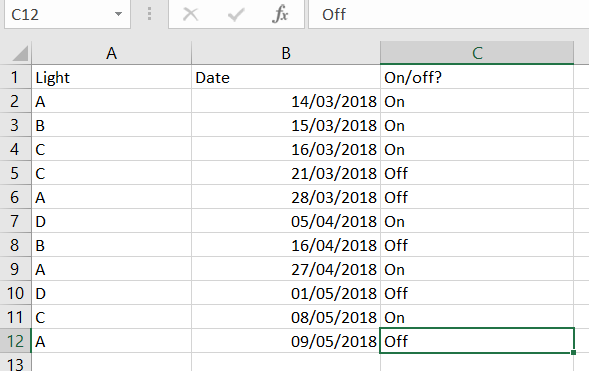Our use case is that we want to find out how many lights were on (or off) for a given date. Notice that we only have dates which show when a light was switched on or off and not the dates in between. Ideally, we want a date for each day in between 14th March and 9th May logging which lights were on. This is where data densification (or more precisely, domain completion) comes into play.

#### Step 1. Convert on/off to 1 or 0

Once taken into tableau the data looks like this: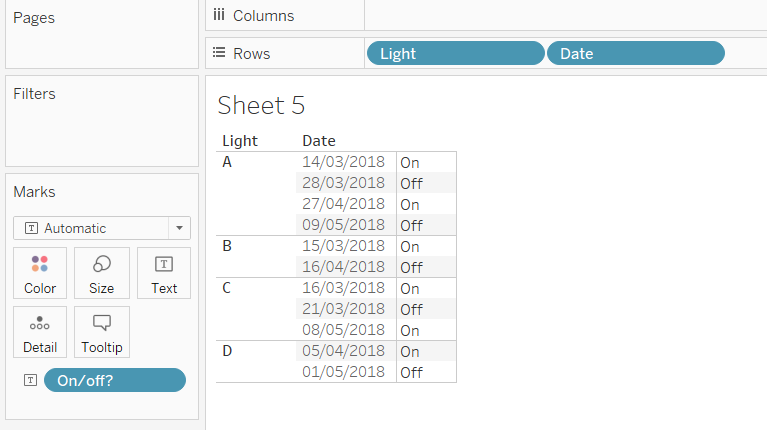At this point we can create a calculation which will convert the on/off to a 1 or 0 using this a simple formula: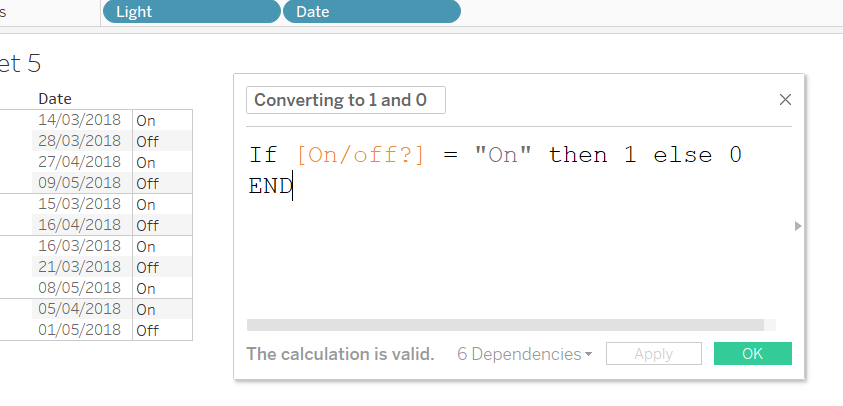#### Step 2. Show missing values

We can re arrange the view so that it looks like this: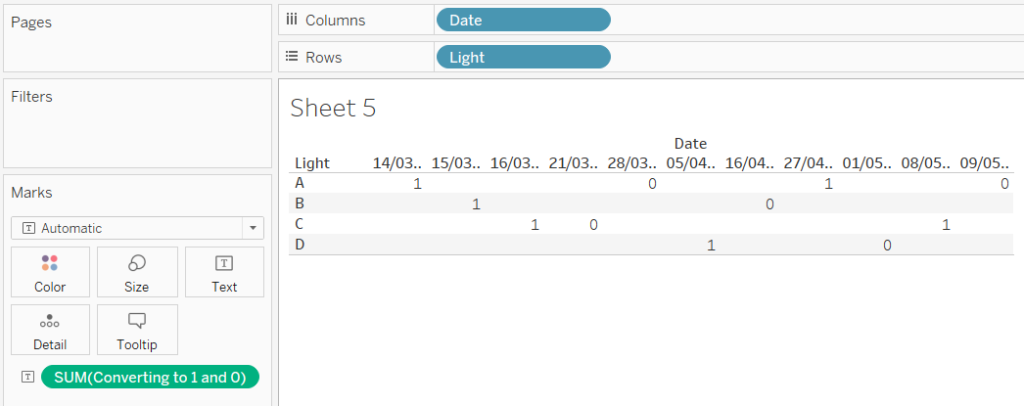As you can see we have a mark for every data entry. See how there are no dates between 16/03 and 21/03. We want the dates in between. To do this, we simply right click on the date pill and select ‘Show missing values’: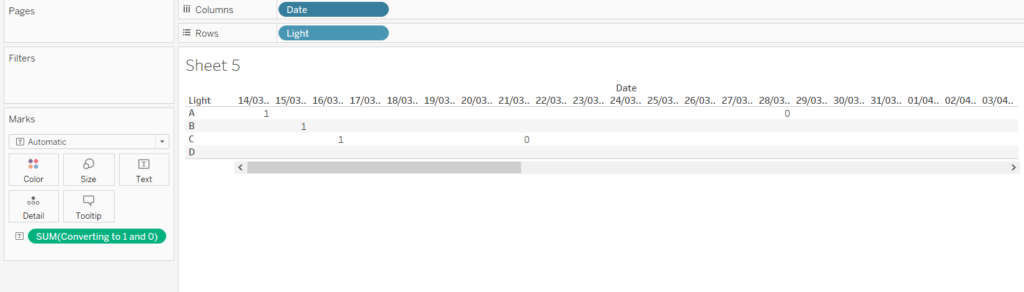This is data densification in action. We have told tableau to show the missing values so that table calculations can now be performed in these missing dates.

Now we need to fill in these missing dates by inserting a 1 or 0 in each box. If a light was switched on, it will remain on until it is switched off. So we need to carry the 1 (or 0) forward until it is switched off or on.

#### Step 3.

The calculation required for carrying the 1 or 0 forward looks like this: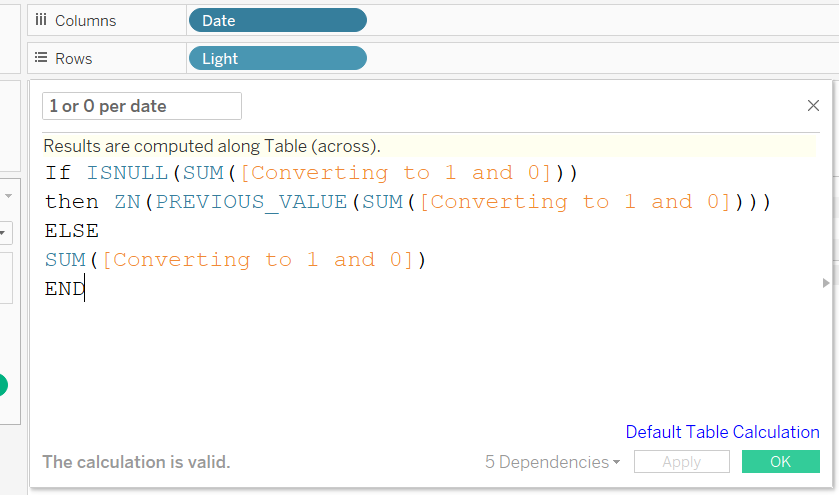The formula basically says that if there is an empty (null) mark then take the previous value. If it is not empty then just give the value. Applying this to our view and making sure that it is calculating across the table we get this: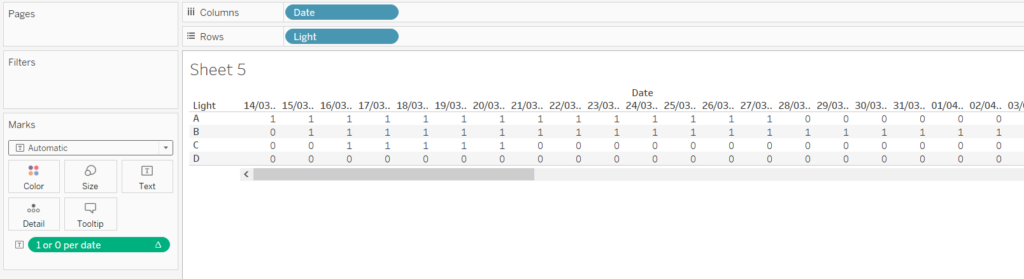#### Step 4.

Next step is to sum the values going down the table for each date. This is another simple formula: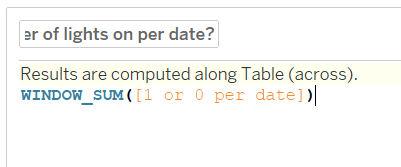Drag this formula to the rows shelf. Because this formula is a nested calculation we must change the way it calculated for the ‘1 or 0 per date’ formula and for the calculation above: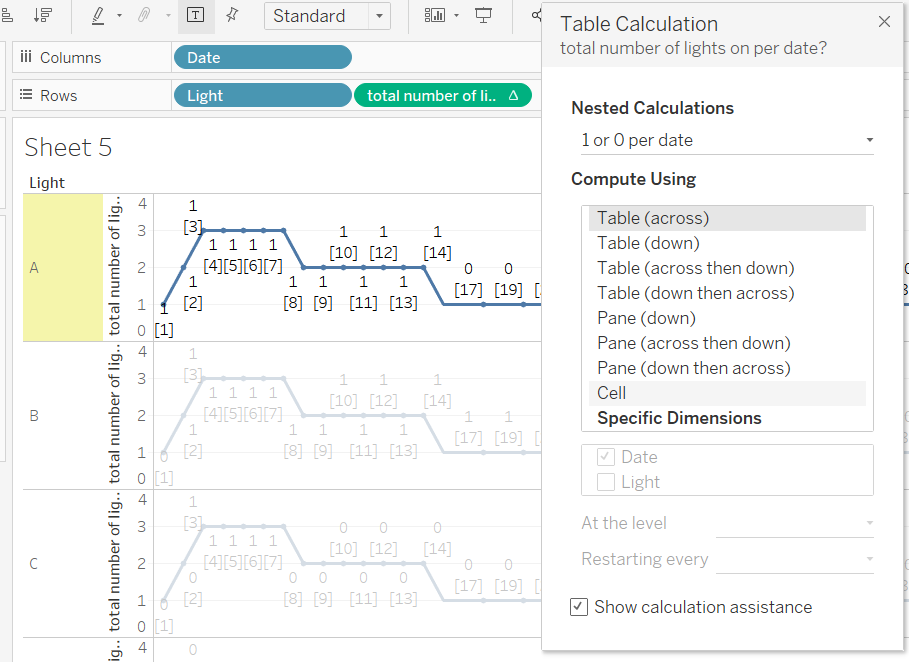Converting the mark type to a line and hiding the B,C and D rows (as they all show the same line chart) we eventually arrive with a chart which shows the number of lights on for every date: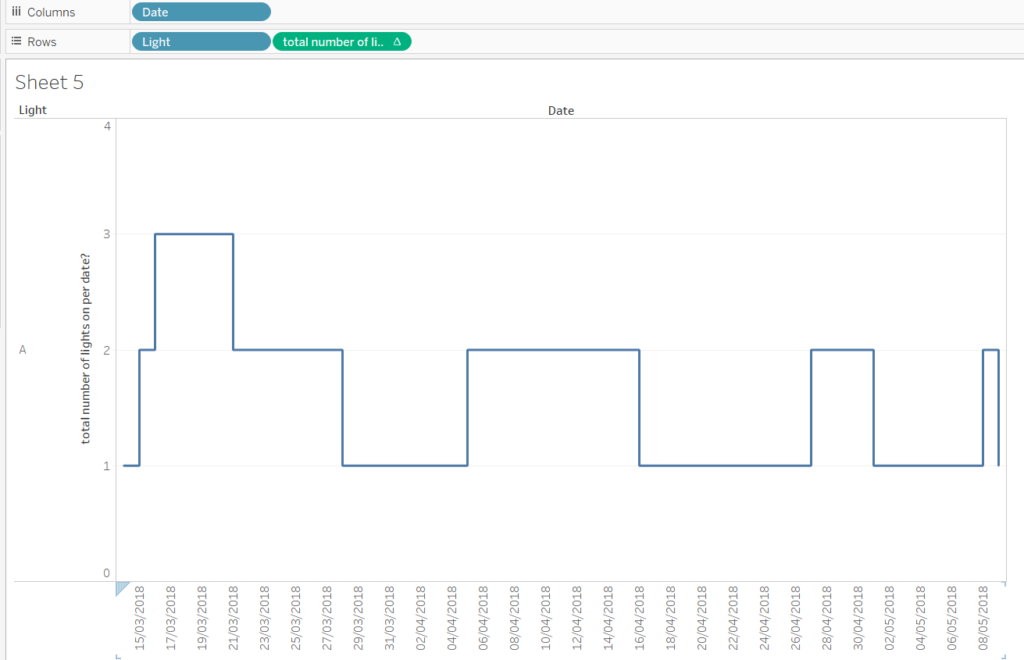Now lets recap what we have done. We initially had 11 rows of data. From this we were able to generate dates in between those dates and come up with a chart which shows the number of lights on for each day. All made possible through data densification. There are many use cases which can benefit from this Tableau feature. So I hope this has been of interest to you, if you have anything you’d like to say to me get in contact using twitter or LinkedIn.Jevon Da Costa